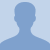# Physics Geometric Optics 10 - Diverging Lens Ray DiagramThis lesson is on diverging lens Ray Diagram. You will learn how to draw a ray diagram for a diverging lens. A diverging lens will be explained and its relation to a convex mirror. A step... This lesson is on diverging lens Ray Diagram. You will learn how to draw a ray diagram for a diverging lens. A diverging lens will be explained and its relation to a convex mirror. A step by step process of how to draw rays and its relation to principal axis, the extension and how it relates to the focus will be shown and explained.
More... Collapse
21 Views